﻿ From order to chaos - Program of course - Education - Saratov group of theoretical nonlinear dynamics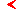main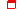this page only

# From order to chaos

Author: Prof. S.P.Kuznetsov

8 lectureseducationproblems and lessons

## 1. Introduction. Scenarios of transition to chaos. General discussion

Problem of transition to chaos under parameter variation. Historical background: Landau and Hopf, Ruelle and Takens, Feigenbaum, Pomeau and Manneville, Arnold and others.

Stability lose of a limit cycle and classification of scenarios of transition to chaos. Linear analysis and "stability triangle". Three ways of typical stability break-up.  Role of nonlinear terms. Scenarios of the onset of chaos via period-doubling, intermittency of type I, II, and III, quasi-periodicity. Problem of the multi-parameter analysis of transitions to chaos and concept of codimension.

## 2. Period-doubling cascade – Feigenbaum scenario: Renormalization group, universality, and scaling

Model system – the logistic map.

Phenomenology of the period-doubling transition to chaos. Bifurcation tree and Lyapunov exponent. Empirical discovery of universal constants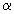and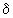by Feigenbaum. Critical point of the period-doubling accumulation and analogy with phase transition theory. Qualitative sketch of dynamics in supercritical domain. Sharkovsky order.

Idea of the renormalization group (RG) analysis. Truncated RG analysis. Unstable periodic orbits at the critical point and universal multiplier.

Derivation of the functional RG equation of Feigenbaum-Cvitanović. Fixed-point solution of the equation. Numerical approach to its computation.

Linearization of the RG equation near the fixed point. Eigenvalue problem. Idea of the numerical solution. Spectrum of eigenvalues. Trivial solutions associated with the variable changes. Saddle nature of the fixed point of the RG equation, interpretation of its stable and unstable manifolds. Explanation of the parameter-space scaling near the critical point. Note concerning dependence of the solution and scaling constants on the order of extremum in the initial map.

## 3. Feigenbaum’s critical attractor

Features of dynamics at the critical point. Critical attractor and its fractal properties. Sigma function. Two-scale Cantor set as a model of the critical attractor. Critical Fourier spectrum and relation of subharmonics of different levels of the hierarchy. Spectrum of Rényi dimensions and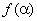-spectrum.

On transition to chaos via period doubling cascade in realistic systems and flow models.

## 4. Intermittency

Intermittency of type I in Lorenz system and in logistic map near a  border of periodicity window. Tangent bifurcation and re-injection condition. Qualitative picture: channel formation, laminar and turbulent stages. Scaling law for duration of the laminar stages in dependence on supercriticality. RG analysis of intermittency by analogy with the Feigenbaum theory. Explicit solution for the RG equation and for the eigenvalue problem

Intermittency of type III. Subcritical period doubling bifurcation and re-injection condition. Qualitative picture: laminar and turbulent stages. Scaling law for duration of the laminar stages in dependence on supercriticality. RG analysis and explicit solution for the RG equation and for the eigenvalue problem

## 5. Quasi-periodic dynamics and transition to chaos in circle map

A problem of bifurcations of two-dimensional torus-attractor. Circle map: subcritical, critical and supercritical cases. Rotation number and its parameter dependence. Periodicity and quasi-periodicity in terms of the rotation number. Chart of dynamical regimes on the parameter plane and Arnold tongues. Devil’s staircase.

Continued fraction representation for the rotation numbers. Rational approximants. Goldem mean and other quadratic irrationals. Fibonacci numbers and rational approximants for the golden mean. A path on the parameter plane of the circle map corresponding to the golden mean rotation number and its terminal – the GM ("golden mean") critical point.

RG analysis at the GM critical point. The main idea: consideration of dynamics at time intervals given by the Fibonacci numbers. Derivation of the RG equation. Idea and results of the numerical solution. Spectrum of the linearized RG equation. How many unstable directions of the fixed point solution are relevant?

Remark on other rotation numbers: structure of the RG equations. A possibility to have fixed points, periodic and non-periodic orbits generated by the RG equations.

Transition to chaos inside the Arnold tongues: period doubling, tricritical points.

## 6. Critical dynamics and scaling properties in the case of golden-mean rotational number

Critical attractor at the GM critical point. Scaling properties in phase space (iteration diagram, invariant measure distribution). Fourier spectrum and its representation in the double logarithmic scale to make visible its basic scaling property.

Parameter space scaling along the critical line for Lyapunov exponent and Devil’s staircase.

Scaling of the Arnold tongues picture near the critical point GM. A necessity of using curvilinear coordinate system ("scaling coordinates") to observe the scaling property.

## Bibliography

1. S.P.Kuznetsov. Dynamical chaos, 2001, Moscow, Fizmatlit (in Russian).
2. H.G.Schuster. Deterministic chaos, 1988, Physik-Verlag.
3. P.Bergé, Y.Pomeau, and C.Vidal. Order from Chaos: Towards a deterministic approach to turbulence, 1986, John Wiley&Sons.
4. Strange attractors (Eds. Ya.G.Sinai and L.P.Shil’nikov), 1981, Moscow, Mir (in Russian).
5. F.C.Moon. Chaotic Vibrations, 1986, John Wiley&Sons.
6. M. J. Feigenbaum. Universal behavior in nonlinear systems. Physica D7, 1983, No 1-3, 16-39.
7. L.D.Landau and E.M.Lifshitz. Hydrodynamics.
8. M. J. Feigenbaum. Universal behavior in nonlinear systems. Physica D7, 1983, No 1-3, 16-39.
9. V.S.Anishchenko. Dynamical Chaos - Models and Experiments. World Scientific, Singapore, 1995.
10. Yu.I.Neimark, P.S.Landa. Stochastic and chaotic oscillations, 1987, Moscow, Nauka (in Russian).
11. M. Schroeder. Fractals, Chaos, Power Laws. Freeman & Co, N.Y., 1991.
12. E.Ott. Chaos in Dynamical Systems. Cambridge Univ.Press, 1993.
13. J.M.T. Thompson and H.B.Stewart. Nonlinear dynamics and chaos. Wiley, N.Y., 1986.
14. C.Beck and F.Schlögl. Thermodynamics of chaotic systems. Cambridge Univ.Press, 1993.
15. J.-P.Eckmann, P.Collet. Iterated Maps as Dynamical Systems, Birkhäuser, Basel, 1980.
16. J. Guckenheimer and P. J. Holmes. Nonlinear oscillations, dynamical systems, and bifurcations of vector fields. Springer-Verlag, Berlin, 1983.maineducationthis page only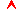top

Saratov group
of theoretical nonlinear
dynamics